#.

This tutorial was authored by Jose A Dianes, PhD.

## Introduction

An important step in data analysis is data exploration and representation. In this tutorial we will see how by combining a technique called Principal Component Analysis (PCA) together with Cluster Analysis we can represent in a two-dimensional space data defined in a higher dimensional one while, at the same time, being able to group this data in similar groups or clusters and find hidden relationships in our data.

More concretely, PCA reduces data dimensionality by finding principal components. These are the directions of maximum variation in a dataset. By reducing a dataset original features or variables to a reduced set of new ones based on the principal components, we end up with the minimum number of variables that keep the maximum amount of variation or information about how the data is distributed. If we end up with just two of these new variables, we will be able to represent each sample in our data in a two-dimensional chart (e.g. a scatterplot).

As an unsupervised data analysis technique, clustering organises data samples by proximity based on its variables. By doing so we will be able to understand how each data point relates to each other and discover groups of similar ones. Once we have each of this groups or clusters, we will be able to define a centroid for them, an ideal data sample that minimises the sum of the distances to each of the data points in a cluster. By analysing these centroids’ variables we will be able to define each cluster in terms of its characteristics.

But enough theory for today. Let’s put these ideas in practice by using Python and R so we better understand them in order to apply them in the future.

All the source code for the different parts of this series of tutorials and applications can be checked at GitHub. Feel free to get involved and share your progress with us!

## Preparing our TB data

We will continue using the same datasets we already loaded in the part introducing data frames. The Gapminder website presents itself as a fact-based worldview. It is a comprehensive resource for data regarding different countries and territories indicators. Its Data section contains a list of datasets that can be accessed as Google Spreadsheet pages (add `&output=csv` to download as CSV). Each indicator dataset is tagged with a Data provider, a Category, and a Subcategory.

For this tutorial, we will use a dataset related to prevalence of Infectious Tuberculosis:

We invite the reader to repeat the process with the new cases and deaths datasets and share the results.

For the sake of making the tutorial self-contained, we will repeat here the code that gets and prepares the datasets both in Python and R. This tutorial is about exploring countries. Therefore, we will work with datasets where each sample is a country and each variable is a year.

### R

In R, you use `read.csv` to read CSV files into `data.frame` variables. Although the R function `read.csv` can work with URLs, https is a problem for R in many cases, so you need to use a package like RCurl to get around it.

``library(RCurl)``
``## Loading required package: bitops``
``````# Get and process existing cases fileexisting_cases_file <-
existing_df <- read.csv(text = existing_cases_file, row.names=1, stringsAsFactor=F)
existing_df[c(1,2,3,4,5,6,15,16,17,18)] <-
lapply( existing_df[c(1,2,3,4,5,6,15,16,17,18)],
function(x) { as.integer(gsub(',', '', x) )})
``````

### Python

``````import urllib

local_tb_existing_file = 'tb_existing_100.csv'
existing_f = urllib.urlretrieve(tb_existing_url_csv,
local_tb_existing_file)
``````

Now that we have it locally, we need to read the CSV file as a data frame.

``````import pandas as pd
local_tb_existing_file,
index_col = 0,
thousands  = ',')
existing_df.index.names = ['country']
existing_df.columns.names = ['year']
``````

We have specified `index_col` to be 0 since we want the country names to be the row labels. We also specified the `thousands` separator to be ‘,’ so Pandas automatically parses cells as numbers. We can use `head()` to check the first few lines.

``existing_df.head()``
year 1990 1991 1992 1993 1994 1995 1996 1997 1998 1999 2000 2001 2002 2003 2004 2005 2006 2007
country
Afghanistan 436 429 422 415 407 397 397 387 374 373 346 326 304 308 283 267 251 238
Albania 42 40 41 42 42 43 42 44 43 42 40 34 32 32 29 29 26 22
Algeria 45 44 44 43 43 42 43 44 45 46 48 49 50 51 52 53 55 56
American Samoa 42 14 4 18 17 22 0 25 12 8 8 6 5 6 9 11 9 5
Andorra 39 37 35 33 32 30 28 23 24 22 20 20 21 18 19 18 17 19

## Dimensionality reduction with PCA

In this section, we want to be able to represent each country in a two dimensional space. In our dataset, each sample is a country defined by 18 different variables, each one corresponding to TB cases counts per 100K (existing, new, deaths) for a given year from 1990 to 2007. These variables represent not just the total counts or average in the 1990-2007 range but also all the variation in the time series and relationships within countries in a given year. By using PCA we will be able to reduce these 18 variables to just the two of them that best captures that information.

In order to do so, we will first how to perform PCA and plot the first two PCs in both, Python and R. We will close the section by analysing the resulting plot and each of the two PCs.

### R

The default R package `stats` comes with function `prcomp()` to perform principal component analysis. This means that we don’t need to install anything (although there are other options using external packages). This is perhaps the quickest way to do a PCA, and I recommend you to call `?prcomp` in your R console if you’re interested in the details of how to fine tune the PCA process with this function.

``pca_existing <- prcomp(existing_df, scale. = TRUE)``

The resulting object contains several pieces of information related with principal component analysis. We are interested in the scores, that we have in `pca_existing\$x`. We got 18 different principal components. Remember that the total number of PCs corresponds to the total number of variables in the dataset, although we normally don’t want to use all of them but the subset that corresponds to our purposes.

In our case we will use the first two. How much variation is explained by each one? In R we can use the `plot` function that comes with the PCA result for that.

``plot(pca_existing)``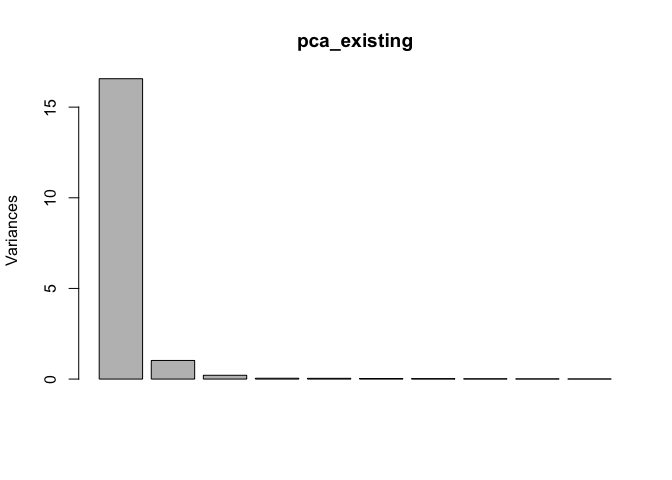Most variation is explained by the first PC. So let’s use the first two PCs to represent all of our countries in a scatterplot.

``````scores_existing_df <- as.data.frame(pca_existing\$x)
# Show first two PCs for head countries
``````
``````##                      PC1          PC2
## Afghanistan    -3.490274  0.973495650
## Albania         2.929002  0.012141345
## Algeria         2.719073 -0.184591877
## American Samoa  3.437263  0.005609367
## Andorra         3.173621  0.033839606
## Angola         -4.695625  1.398306461
``````

Now that we have them in a data frame, we can use them with `plot`.

``````plot(PC1~PC2, data=scores_existing_df, main= "Existing TB cases per 100K distribution",
cex = .1, lty = "solid")
text(PC1~PC2, data=scores_existing_df,
labels=rownames(existing_df),
cex=.8)
``````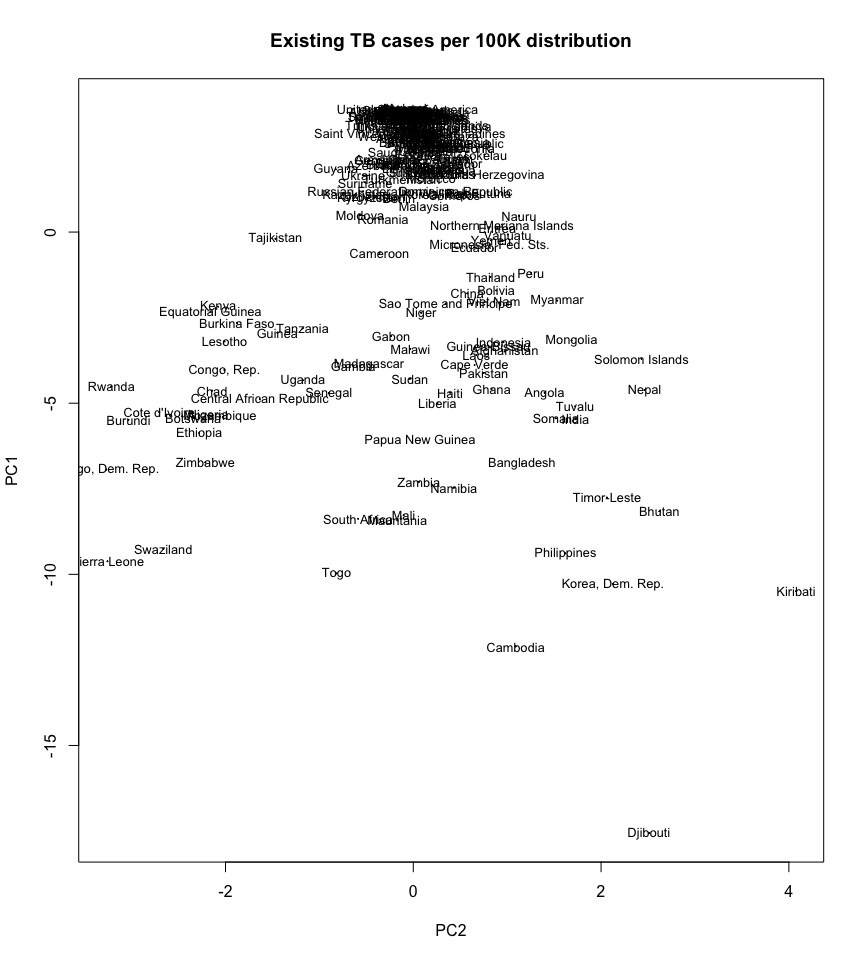Let’s set the color associated with the mean value for all the years. We will use functions `rgb`, `ramp`, and `rescale` to create a color palette from yellow (lower values) to blue (higher values).

``````library(scales)ramp <- colorRamp(c("yellow", "blue"))colours_by_mean <- rgb(
ramp( as.vector(rescale(rowMeans(existing_df),c(0,1)))),
max = 255 )
plot(PC1~PC2, data=scores_existing_df,
main= "Existing TB cases per 100K distribution",
cex = .1, lty = "solid", col=colours_by_mean)
text(PC1~PC2, data=scores_existing_df,
labels=rownames(existing_df),
cex=.8, col=colours_by_mean)
``````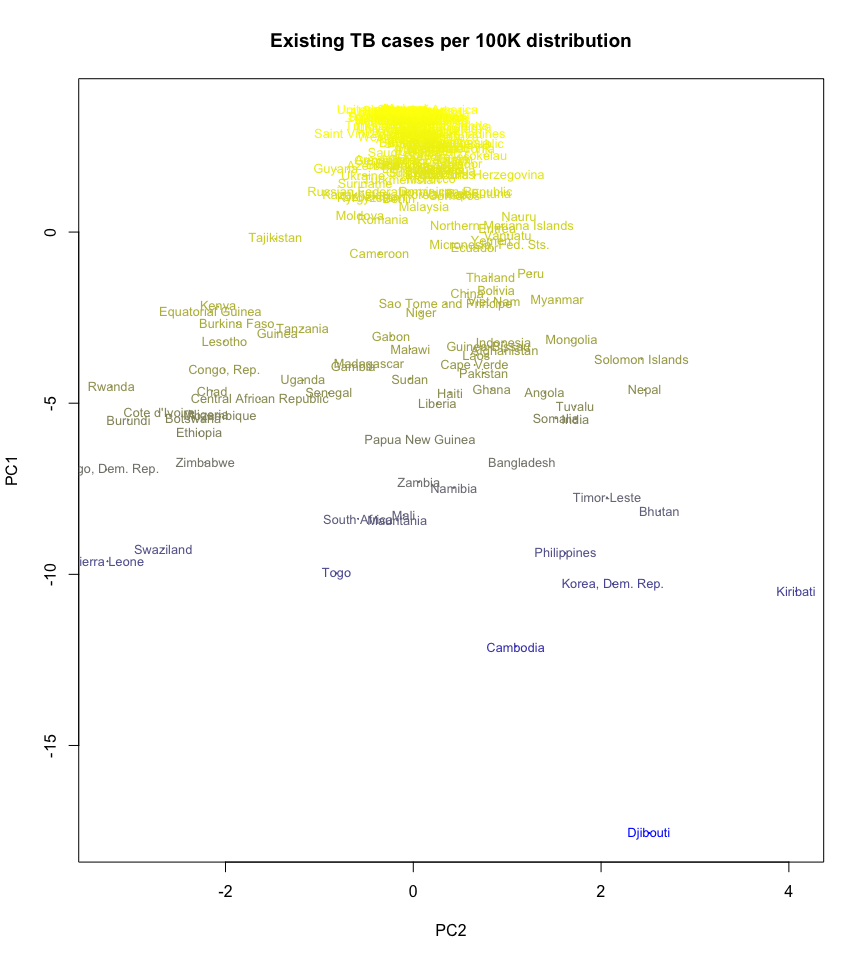Now let’s associate colour with total sum.

``````ramp <- colorRamp(c("yellow", "blue"))colours_by_sum <- rgb(
ramp( as.vector(rescale(rowSums(existing_df),c(0,1)))),
max = 255 )
plot(PC1~PC2, data=scores_existing_df,
main= "Existing TB cases per 100K distribution",
cex = .1, lty = "solid", col=colours_by_sum)
text(PC1~PC2, data=scores_existing_df,
labels=rownames(existing_df),
cex=.8, col=colours_by_sum)
``````And finally let’s associate it with the difference between first and last year, as a simple way to measure the change in time.

``````existing_df_change <- existing_df\$X2007 - existing_df\$X1990ramp <- colorRamp(c("yellow", "blue"))
colours_by_change <- rgb(
ramp( as.vector(rescale(existing_df_change,c(0,1)))),
max = 255 )
plot(PC1~PC2, data=scores_existing_df,
main= "Existing TB cases per 100K distribution",
cex = .1, lty = "solid", col=colours_by_change)
text(PC1~PC2, data=scores_existing_df,
labels=rownames(existing_df),
cex=.8, col=colours_by_change)
``````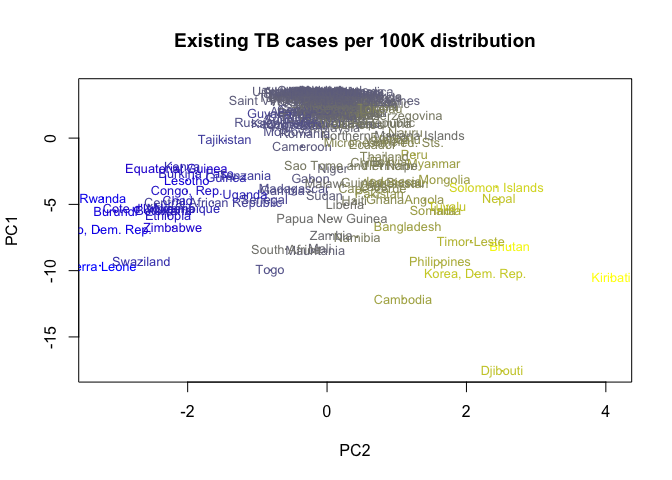We have some interesting conclusions already about what PC1 and PC2 code as a representation of the years from 1990 to 2008. We will explain that right after showing how to perform dimensionality reduction using Python.

### Python

Python’s sklearn machine learning library comes with a [PCA implementation](http ://scikit-learn.org/stable/modules/generated/sklearn.decomposition.PCA.html). This implementation uses the `scipy.linalg` implementation of the singular value decomposition. It only works for dense arrays (see numPy dense arrays or sparse array PCA if you are using sparse arrays) and is not scalable to large dimensional data. For large dimensional data we should consider something such as Spark’s dimensionality reduction features. In our case we just have 18 variables, and that is far from being a large number of features for today’s machine learning libraries and computer capabilities.

When using this implementation of PCA we need to specify in advance the number of principal components we want to use. Then we can just call the `fit()` method with our data frame and check the results.

``````from sklearn.decomposition import PCA
pca = PCA(n_components=2)
pca.fit(existing_df)
``````
``PCA(copy=True, n_components=2, whiten=False)``

This gives us an object we can use to transform our data by calling `transform`.

``existing_2d = pca.transform(existing_df)``

Or we could have just called `fit_transform` to perform both steps in one single call.

In both cases we will end up with a lower dimension representation of our data frame, as a numPy array. Let’s put it in a new data frame.

``````existing_df_2d = pd.DataFrame(existing_2d)existing_df_2d.index = existing_df.index
existing_df_2d.columns = ['PC1','PC2']
``````
PC1 PC2
country
Afghanistan -732.215864 203.381494
Albania 613.296510 4.715978
Algeria 569.303713 -36.837051
American Samoa 717.082766 5.464696
Andorra 661.802241 11.037736

We can also print the explained variance ratio as follows.

``print(pca.explained_variance_ratio_)``
``[ 0.91808789  0.060556  ]``

We see that the first PC already explains almost 92% of the variance, while the second one accounts for another 6% for a total of almost 98% between the two of them.

Now we are ready to plot the lower dimensionality version of our dataset. We just need to call plot on the data frame, by passing the kind of plot we want (see here for more on plotting data frames) and what columns correspond to each axis. We also add an annotation loop that tags every point with its country name.

``````%matplotlib inline    ax = existing_df_2d.plot(kind='scatter', x='PC2', y='PC1', figsize=(16,8))

for i, country in enumerate(existing_df.index):
ax.annotate(
country,
(existing_df_2d.iloc[i].PC2, existing_df_2d.iloc[i].PC1)
)
``````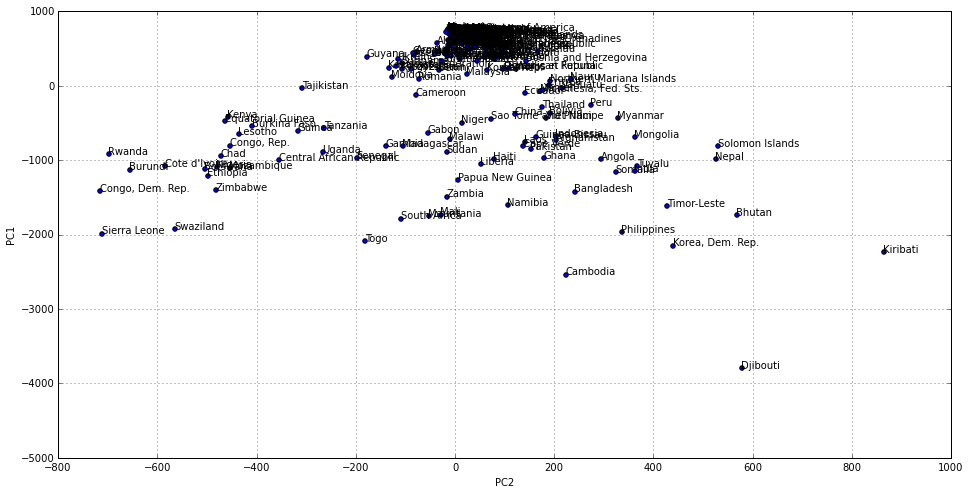Let’s now create a bubble chart, by setting the point size to a value proportional to the mean value for all the years in that particular country. First we need to add a new column containing the re-scaled mean per country across all the years.

``````from sklearn.preprocessing import normalize
existing_df_2d['country_mean'] = pd.Series(existing_df.mean(axis=1), index=existing_df_2d.index)
country_mean_max = existing_df_2d['country_mean'].max()
country_mean_min = existing_df_2d['country_mean'].min()
country_mean_scaled =
(existing_df_2d.country_mean-country_mean_min) / country_mean_max
existing_df_2d['country_mean_scaled'] = pd.Series(
country_mean_scaled,
index=existing_df_2d.index)

``````
PC1 PC2 country_mean country_mean_scaled
country
Afghanistan -732.215864 203.381494 353.333333 0.329731
Albania 613.296510 4.715978 36.944444 0.032420
Algeria 569.303713 -36.837051 47.388889 0.042234
American Samoa 717.082766 5.464696 12.277778 0.009240
Andorra 661.802241 11.037736 25.277778 0.021457

Now we are ready to plot using this variable size (we will omit the country names this time since we are not so interested in them).

``````existing_df_2d.plot(kind='scatter',
x='PC2',
y='PC1',
s=existing_df_2d['country_mean_scaled']*100,
figsize=(16,8))
``````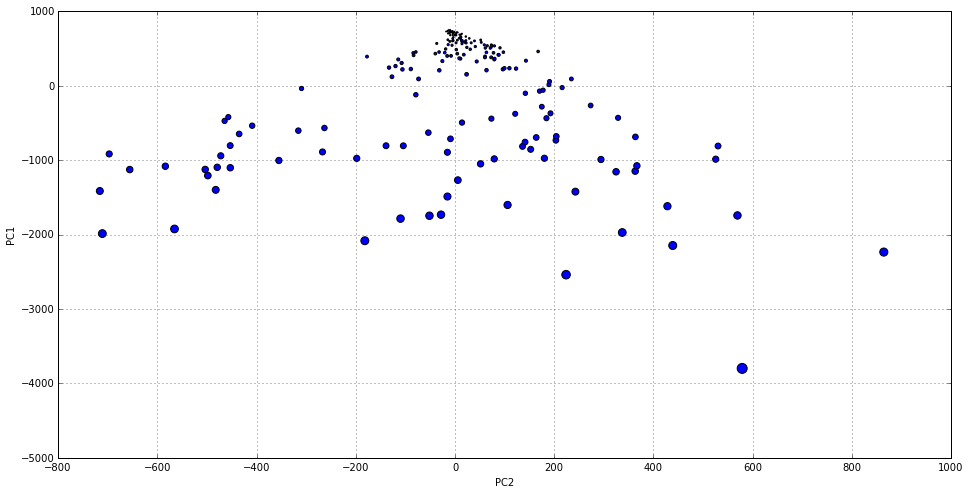Let’s do the same with the sum instead of the mean.

``````existing_df_2d['country_sum'] = pd.Series(existing_df.sum(axis=1),
index=existing_df_2d.index)
country_sum_max = existing_df_2d['country_sum'].max()
country_sum_min = existing_df_2d['country_sum'].min()
country_sum_scaled =
(existing_df_2d.country_sum-country_sum_min) / country_sum_max
existing_df_2d['country_sum_scaled'] = pd.Series(
country_sum_scaled,
index=existing_df_2d.index)
existing_df_2d.plot(
kind='scatter',
x='PC2', y='PC1',
s=existing_df_2d['country_sum_scaled']*100,
figsize=(16,8))
``````And finally let’s associate the size with the change between 1990 and 2007. Note that in the scaled version, those values close to zero will make reference to those with negative values in the original non-scaled version, since we are scaling to a [0,1] range.

``````existing_df_2d['country_change'] = pd.Series(existing_df['2007']-existing_df['1990'],
index=existing_df_2d.index)
country_change_max = existing_df_2d['country_change'].max()
country_change_min = existing_df_2d['country_change'].min()
country_change_scaled =
(existing_df_2d.country_change - country_change_min) / country_change_max
existing_df_2d['country_change_scaled'] = pd.Series(
country_change_scaled,
index=existing_df_2d.index)
``````
country_change country_change_scaled
country
Afghanistan -198 0.850840
Albania -20 1.224790
Algeria 11 1.289916
American Samoa -37 1.189076
Andorra -20 1.224790
``````existing_df_2d.plot(
kind='scatter',
x='PC2', y='PC1',
s=existing_df_2d['country_change_scaled']*100,
figsize=(16,8))
``````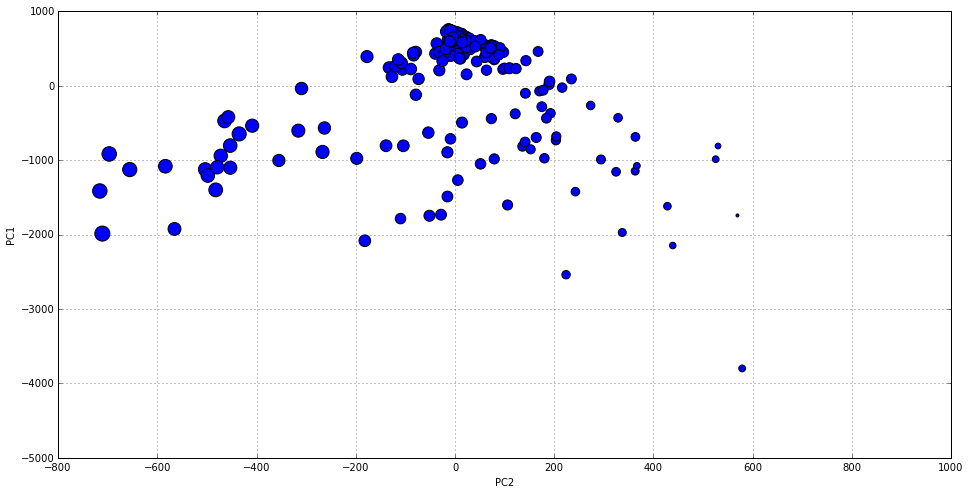### PCA Results

From the plots we have done in Python and R, we can confirm that the most variation happens along the y axis, which we have assigned to PC1. We saw that the first PC already explains almost 92% of the variance, while the second one accounts for another 6% for a total of almost 98% between the two of them. At the very top of our charts we saw an important concentration of countries, most of them developed. While we descend that axis, the number of countries is more sparse, and they belong to less developed regions of the world.

When colouring/sizing points using two absolute magnitudes such as average and total number of cases, we can see that the directions also correspond to a variation in these magnitudes.

Moreover, when using color/size to code the difference in the number of cases over time (2007 minus 1990), the color gradient mostly changed along the direction of the second principal component, with more positive values (i.e. increase in the number of cases) coloured in blue or with bigger size . That is, while the first PC captures most of the variation within our dataset and this variation is based on the total cases in the 1990-2007 range, the second PC is largely affected by the change over time.

In the next section we will try to discover other relationships between countries.

## Exploring data structure with k-means clustering

In this section we will use k-means clustering to group countries based on how similar their situation has been year-by-year. That is, we will cluster the data based in the 18 variables that we have. Then we will use the cluster assignment to colour the previous 2D chart, in order to discover hidden relationship within our data and better understand the world situation regarding the tuberculosis disease.

When using k-means, we need to determine the right number of groups for our case. This can be done more or less accurately by iterating through different values for the number of groups and compare an amount called the within-cluster sum of square distances for each iteration. This is the squared sum of distances to the cluster center for each cluster member. Of course this distance is minimal when the number of clusters gets equal to the number of samples, but we don’t want to get there. We normally stop when the improvement in this value starts decreasing at a lower rate.

However, we will use a more intuitive approach based on our understanding of the world situation and the nature of the results that we want to achieve. Sometimes this is the way to go in data analysis, specially when doing exploration tasks. To use the knowledge that we have about the nature of our data is always a good thing to do.

### R

Obtaining clusters in R is as simple as calling to `kmeans`. The function has several parameters, but we will just use all the defaults and start trying with different values of k.

Let’s start with `k=3` asuming that at least, the are countries in a really bad situation, countries in a good situation, and some of them in between.

``set.seed(1234)existing_clustering <- kmeans(existing_df, centers = 3)``

The result contains a list with components:

• `cluster`: A vector of integers indicating the cluster to which each point is allocated.
• `centers`: A matrix of cluster centres.
• `withinss`: The within-cluster sum of square distances for each cluster.
• `size`: The number of points in each cluster.

Let’s colour our previous scatter plot based on what cluster each country belongs to.

``````existing_cluster_groups <- existing_clustering\$clusterplot(PC1~PC2, data=scores_existing_df,
main= "Existing TB cases per 100K distribution",
cex = .1, lty = "solid", col=existing_cluster_groups)
text(PC1~PC2, data=scores_existing_df,
labels=rownames(existing_df),
cex=.8, col=existing_cluster_groups)
``````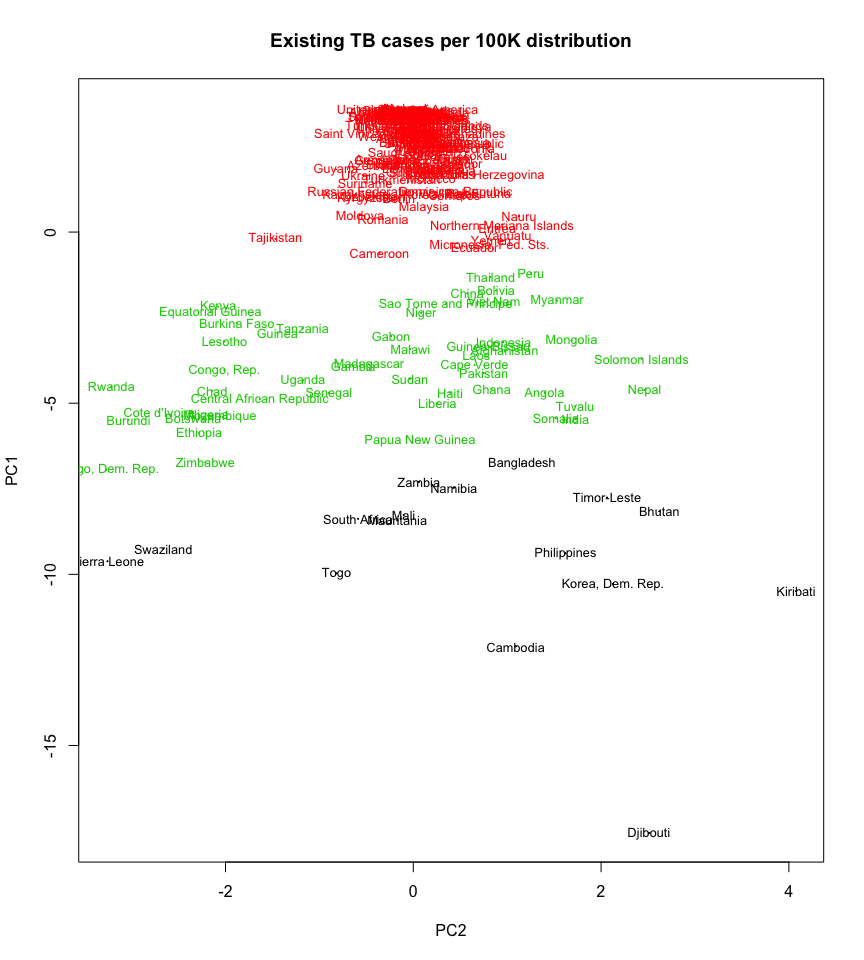Most clusters are based on the first PC. That means that clusters are just defined in terms of the total number of cases per 100K and not how the data evolved on time (PC2). So let’s try with `k=4` and see if some of these cluster are refined in the direction of the second PC.

``````set.seed(1234)existing_clustering <- kmeans(existing_df, centers = 4)
existing_cluster_groups <- existing_clustering\$cluster
plot(PC1~PC2, data=scores_existing_df,
main= "Existing TB cases per 100K distribution",
cex = .1, lty = "solid", col=existing_cluster_groups)
text(PC1~PC2, data=scores_existing_df,
labels=rownames(existing_df),
cex=.8, col=existing_cluster_groups)
``````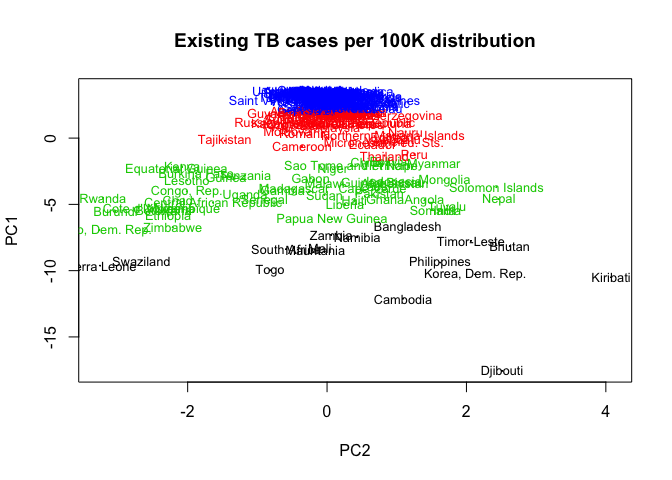There is more refinement, but again in the direction of the first PC. Let’s try then with `k=5`.

``````set.seed(1234)existing_clustering <- kmeans(existing_df, centers = 5)
existing_cluster_groups <- existing_clustering\$cluster
plot(PC1~PC2, data=scores_existing_df,
main= "Existing TB cases per 100K distribution",
cex = .1, lty = "solid", col=existing_cluster_groups)
text(PC1~PC2, data=scores_existing_df,
labels=rownames(existing_df),
cex=.8, col=existing_cluster_groups)
``````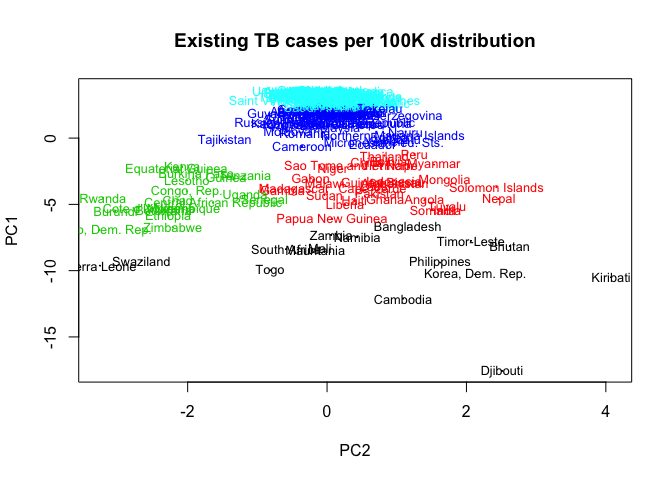There we have it. Right in the middle we have a cluster that has been split in two different ones in the direction of the second PC. What if we try with `k=6`?

``````set.seed(1234)existing_clustering <- kmeans(existing_df, centers = 6)
existing_cluster_groups <- existing_clustering\$cluster
plot(PC1~PC2, data=scores_existing_df,
main= "Existing TB cases per 100K distribution",```
cex = .1, lty = "solid", col=existing_cluster_groups)
text(PC1~PC2, data=scores_existing_df,
labels=rownames(existing_df),
cex=.8, col=existing_cluster_groups)
```

`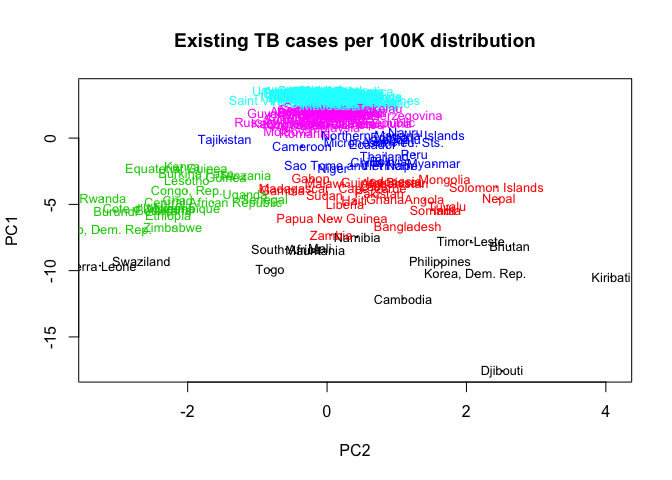`

`We get some diagonal split in the second top cluster. That surely contains some interesting information, but let’s revert to our k=5 case and later on we will see how to use a different refinement process with clusters are too tight like we have at the top of the plot.`

``````set.seed(1234)existing_clustering <- kmeans(existing_df, centers = 5)
existing_cluster_groups <- existing_clustering\$cluster```
plot(PC1~PC2, data=scores_existing_df,
main= "Existing TB cases per 100K distribution",
cex = .1, lty = "solid", col=existing_cluster_groups)
text(PC1~PC2, data=scores_existing_df,
labels=rownames(existing_df),
cex=.8, col=existing_cluster_groups)
```

``

### `Python`

`Again we will use sklearn, in this case its k-means clustering implementation, in order to perform our clustering on the TB data. Since we already decided on a number of clusters of 5, we will use it here straightaway.`

``````from sklearn.cluster import KMeans
kmeans = KMeans(n_clusters=5)
clusters = kmeans.fit(existing_df)
``````

Now we need to store the cluster assignments together with each country in our data frame. The cluster labels are returned in `clusters.labels_`.

``existing_df_2d['cluster'] = pd.Series(clusters.labels_, index=existing_df_2d.index)``

And now we are ready to plot, using the cluster column as color.

``````import numpy as np
existing_df_2d.plot(
kind='scatter',
x='PC2',y='PC1',
c=existing_df_2d.cluster.astype(np.float),
figsize=(16,8))
``````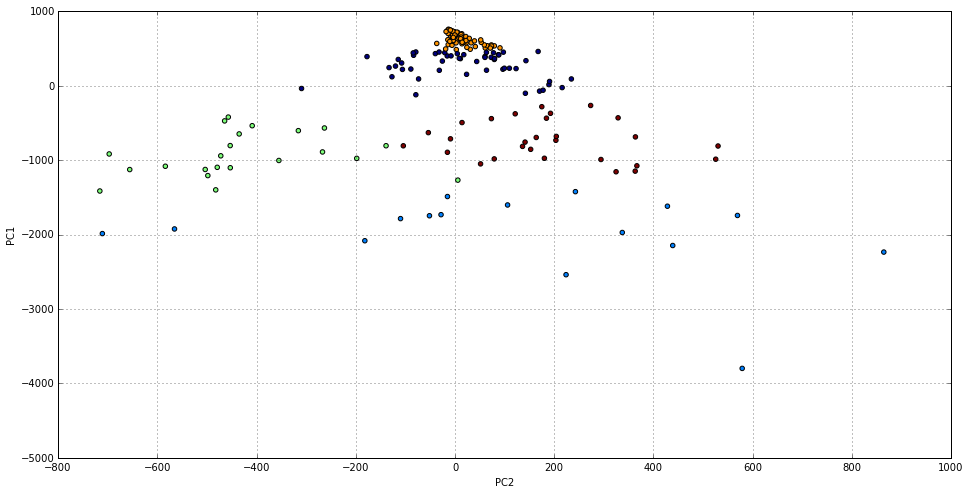The result is pretty much as the one obtained with R, with the color differences and without the country names that we decided not to include here so we can better see the colours. In the next section we will analyse each cluster in detail.

## Cluster interpretation

Most of the work in this section is about data frame indexing and plotting. There isn’t anything sophisticated about the code itself, so we will pick up one of our languages and perform the whole thing (we will use R this time).

In order to analyse each cluster, let’s add a column in our data frame containing the cluster ID. We will use that for subsetting.

``````existing_df\$cluster <- existing_clustering\$clustertable(existing_df\$cluster)

##
##  1  2  3  4  5
## 16 30 20 51 90
``````

The last line shows how many countries do we have in each cluster.

### Centroids comparison chart

Let’s start by creating a line chart that compares the time series for each cluster centroid. This chart will helps us better understand our cluster results.

``````xrange <- 1990:2007plot(xrange, existing_clustering\$centers[1,],
type='l', xlab="Year",

ylab="New cases per 100K",

col = 1,
ylim=c(0,1000))
for (i in 2:nrow(existing_clustering\$centers)) {
lines(xrange, existing_clustering\$centers[i,],
col = i)
}
legend(x=1990, y=1000,
lty=1, cex = 0.5,
ncol = 5,
col=1:(nrow(existing_clustering\$centers)+1),
legend=paste("Cluster",1:nrow(existing_clustering\$centers)))
``````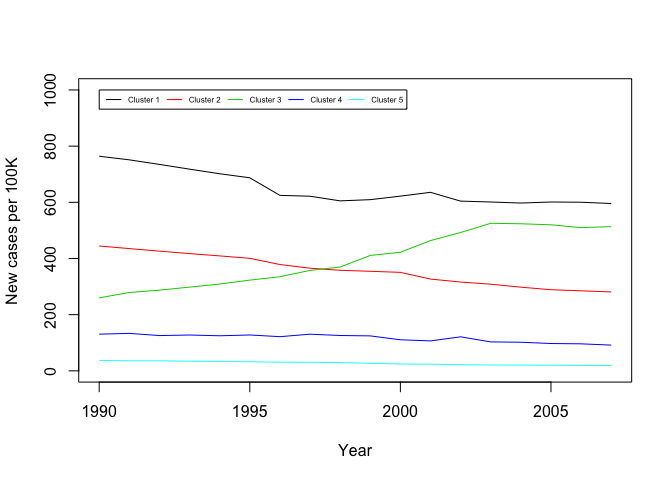### Cluster 1

Cluster 1 contains just 16 countries. These are:

``rownames(subset(existing_df, cluster==1))``
``````##   "Bangladesh"       "Bhutan"           "Cambodia"##   "Korea, Dem. Rep." "Djibouti"         "Kiribati"
##   "Mali"             "Mauritania"       "Namibia"
##  "Philippines"      "Sierra Leone"     "South Africa"
##  "Swaziland"        "Timor-Leste"      "Togo"
##  "Zambia"
``````

The centroid that represents them is:

``existing_clustering\$centers[1,]``
``````##    X1990    X1991    X1992    X1993    X1994    X1995    X1996    X1997
## 764.0000 751.1875 734.9375 718.0625 701.6875 687.3125 624.7500 621.6250

##    X1998    X1999    X2000    X2001    X2002    X2003    X2004    X2005
## 605.1875 609.4375 622.0000 635.5000 604.2500 601.1250 597.3750 601.1250
##    X2006    X2007
## 600.2500 595.7500
``````

These are by all means the countries with the most tuberculosis cases every year. We can see in the chart that this is the top line, although the number of cases descends progressively.

### Cluster 2

Cluster 2 contains 30 countries. These are:

``rownames(subset(existing_df, cluster==2))``
``````##   "Afghanistan"           "Angola"
##   "Bolivia"               "Cape Verde"
##   "China"                 "Gabon"
##   "Gambia"                "Ghana"
##   "Guinea-Bissau"         "Haiti"
##  "India"                 "Indonesia"
##  "Laos"                  "Liberia"
##  "Mongolia"              "Myanmar"
##  "Nepal"                 "Niger"
##  "Pakistan"              "Papua New Guinea"
##  "Peru"                  "Sao Tome and Principe"
##  "Solomon Islands"       "Somalia"
##  "Sudan"                 "Thailand"
##  "Tuvalu"                "Viet Nam"
``````

The centroid that represents them is:

``existing_clustering\$centers[2,]``
``````##    X1990    X1991    X1992    X1993    X1994    X1995    X1996    X1997## 444.5000 435.2000 426.1667 417.4000 409.2333 400.5667 378.6000 365.3667
##    X1998    X1999    X2000    X2001    X2002    X2003    X2004    X2005
## 358.0333 354.4333 350.6000 326.7333 316.1667 308.5000 297.8667 288.8000
##    X2006    X2007
## 284.9667 280.8000
``````

It is a relatively large cluster. Still countries with lots of cases, but definitively less than the first cluster. We see countries such as India or China here, the larger countries on earth (from a previous tutorial we know that China itself has reduced its cases by 85%) and american countries such as Peru or Bolivia. In fact, this is the cluster with the fastest decrease in the number of existing cases as we see in the line chart.

### Cluster 3

This is an important one. Cluster 3 contains just 20 countries. These are:

``rownames(subset(existing_df, cluster==3))``
``````##   "Botswana"                 "Burkina Faso"            ##   "Burundi"                  "Central African Republic"
##   "Cote d'Ivoire"            "Congo, Dem. Rep."
##   "Equatorial Guinea"        "Ethiopia"
##  "Guinea"                   "Kenya"
##  "Lesotho"                  "Mozambique"
##  "Nigeria"                  "Rwanda"
##  "Senegal"                  "Uganda"
##  "Tanzania"                 "Zimbabwe"
``````

The centroid that represents them is:

``existing_clustering\$centers[3,]``
``````##  X1990  X1991  X1992  X1993  X1994  X1995  X1996  X1997  X1998  X1999 ## 259.85 278.90 287.30 298.05 309.00 322.95 335.00 357.65 369.65 410.85

##  X2000  X2001  X2002  X2003  X2004  X2005  X2006  X2007
## 422.25 463.75 492.45 525.25 523.60 519.90 509.80 513.50
``````

This is the only cluster where the number of cases has increased over the years, and is about to overtake the first position by 2007. Each of these countries are probably in the middle of an humanitarian crisis and probably being affected by other infectious diseases such as HIV. We can confirm here that PC2 is coding mostly that, the percentage of variation over time of the number of exiting cases.

### Cluster 4

The fourth cluster contains 51 countries.

``rownames(subset(existing_df, cluster==4))``
``````##   "Armenia"                  "Azerbaijan"              ##   "Bahrain"                  "Belarus"
##   "Benin"                    "Bosnia and Herzegovina"
##   "Brazil"                   "Brunei Darussalam"
##   "Cameroon"                 "Comoros"
##  "Croatia"                  "Dominican Republic"
##  "Eritrea"                  "Georgia"
##  "Guam"                     "Guatemala"
##  "Guyana"                   "Honduras"
##  "Iraq"                     "Kazakhstan"
##  "Kyrgyzstan"               "Latvia"
##  "Lithuania"                "Malaysia"
##  "Maldives"                 "Micronesia, Fed. Sts."
##  "Morocco"                  "Nauru"
##  "Nicaragua"                "Niue"
##  "Northern Mariana Islands" "Palau"
##  "Paraguay"                 "Qatar"
##  "Korea, Rep."              "Moldova"
##  "Romania"                  "Russian Federation"
##  "Seychelles"               "Sri Lanka"
##  "Suriname"                 "Tajikistan"
##  "Tokelau"                  "Turkmenistan"
##  "Ukraine"                  "Uzbekistan"
##  "Vanuatu"                  "Wallis et Futuna"
##  "Yemen"
``````

Represented by its centroid.

``existing_clustering\$centers[4,]``
``````##     X1990     X1991     X1992     X1993     X1994     X1995     X1996 ## 130.60784 133.41176 125.60784 127.54902 124.82353 127.70588 121.68627
##     X1997     X1998     X1999     X2000     X2001     X2002     X2003
## 130.50980 125.82353 124.45098 110.58824 106.60784 121.09804 103.01961
##     X2004     X2005     X2006     X2007
## 101.80392  97.29412  96.17647  91.68627
``````

This cluster is pretty close to the last and larger one. It contains many American countries, some European countries, etc. Some of them are large and rich, such as Russia or Brazil. Structurally the difference with the countries in Cluster 5 may reside in a larger number of cases per 100K. They also seem to be decreasing the number of cases slightly faster than Cluster 5. These two reasons made k-means cluster them in a different group.

### Cluster 5

The last and bigger cluster contains 90 countries.

``rownames(subset(existing_df, cluster==5))``
``````##   "Albania"                          "Algeria"                         ##   "American Samoa"                   "Andorra"

##   "Anguilla"                         "Antigua and Barbuda"
##   "Argentina"                        "Australia"
##   "Austria"                          "Bahamas"
##  "Belize"                           "Bermuda"
##  "British Virgin Islands"           "Bulgaria"
##  "Chile"                            "Colombia"
##  "Cook Islands"                     "Costa Rica"
##  "Cuba"                             "Cyprus"
##  "Czech Republic"                   "Denmark"
##  "Dominica"                         "Egypt"
##  "Estonia"                          "Fiji"
##  "Finland"                          "France"
##  "French Polynesia"                 "Germany"
##  "Hungary"                          "Iceland"
##  "Iran"                             "Ireland"
##  "Israel"                           "Italy"
##  "Jamaica"                          "Japan"
##  "Jordan"                           "Kuwait"
##  "Lebanon"                          "Libyan Arab Jamahiriya"
##  "Luxembourg"                       "Malta"
##  "Mauritius"                        "Mexico"
##  "Monaco"                           "Montserrat"
##  "Netherlands"                      "Netherlands Antilles"
##  "New Caledonia"                    "New Zealand"
##  "Norway"                           "Oman"
##  "Panama"                           "Poland"
##  "Portugal"                         "Puerto Rico"
##  "Saint Kitts and Nevis"            "Saint Lucia"
##  "Saint Vincent and the Grenadines" "Samoa"
##  "San Marino"                       "Saudi Arabia"
##  "Singapore"                        "Slovakia"
##  "Slovenia"                         "Spain"
##  "Sweden"                           "Switzerland"
##  "Syrian Arab Republic"             "Macedonia, FYR"
##  "Tonga"                            "Trinidad and Tobago"
##  "Tunisia"                          "Turkey"
##  "Turks and Caicos Islands"         "United Arab Emirates"
##  "United Kingdom"                   "Virgin Islands (U.S.)"
##  "United States of America"         "Uruguay"
##  "Venezuela"                        "West Bank and Gaza"
``````

Represented by its centroid.

``existing_clustering\$centers[5,]``
``````##    X1990    X1991    X1992    X1993    X1994    X1995    X1996    X1997
## 37.27778 35.68889 35.73333 34.40000 33.51111 32.42222 30.80000 30.51111

##    X1998    X1999    X2000    X2001    X2002    X2003    X2004    X2005
## 29.30000 26.77778 24.35556 23.57778 22.02222 20.93333 20.48889 19.92222
##    X2006    X2007
## 19.25556 19.11111

``````

This cluster is too large and heterogeneous and probably needs further refinement. However, it is a good grouping when compared to other distant clusters. In any case it contains those countries with less number of existing cases in our set.

### A second level of clustering

So let’s do just that quickly. Let’s re-cluster the 90 countries in our Cluster 5 in order to firther refine them. As the number of clusters let’s use 2. We are just interested in seeing if there are actually two different clusters withing Cluster 5. The reader can of course try to go further and use more than 2 centers.

``````# subset the original datasetcluster5_df <- subset(existing_df, cluster==5)

# do the clustering
set.seed(1234)
cluster5_clustering <- kmeans(cluster5_df[,-19], centers = 2)
# assign sub-cluster number to the data set for Cluster 5
cluster5_df\$cluster <- cluster5_clustering\$cluster
``````

Now we can plot them in order to see if there are actual differences.

``````xrange <- 1990:2007plot(xrange, cluster5_clustering\$centers[1,],type='l', xlab="Year",
ylab="Existing cases per 100K",
col = 1,
ylim=c(0,200))
for (i in 2:nrow(cluster5_clustering\$centers)) {
lines(xrange, cluster5_clustering\$centers[i,],
col = i)
}
legend(x=1990, y=200,
lty=1, cex = 0.5,
ncol = 5,
col=1:(nrow(cluster5_clustering\$centers)+1),
legend=paste0("Cluster
5.",1:nrow(cluster5_clustering\$centers)))
``````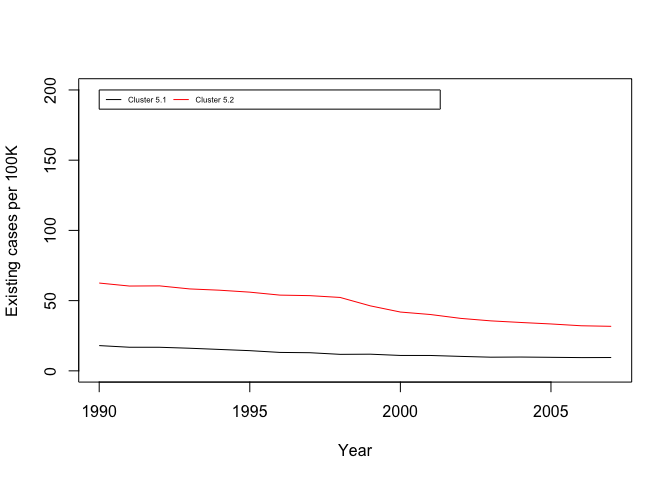There are actually different tendencies in our data. We can see that there is a group of countries in our original Cluster 5 that is decreasing the number cases at a faster rate, trying to catch up with those countries with a lower number of existing TB cases per 100K.

``rownames(subset(cluster5_df, cluster5_df\$cluster==2))``
``````##   "Albania"                          "Algeria"                         ##   "Anguilla"                         "Argentina"
##   "Bahamas"                          "Belize"
##   "Bulgaria"                         "Colombia"
##   "Egypt"                            "Estonia"
##  "Fiji"                             "French Polynesia"
##  "Hungary"                          "Iran"
##  "Japan"                            "Kuwait"
##  "Lebanon"                          "Libyan Arab Jamahiriya"
##  "Mauritius"                        "Mexico"
##  "New Caledonia"                    "Panama"
##  "Poland"                           "Portugal"
##  "Saint Vincent and the Grenadines" "Samoa"
##  "Saudi Arabia"                     "Singapore"
##  "Slovakia"                         "Slovenia"
##  "Spain"                            "Syrian Arab Republic"
##  "Macedonia, FYR"                   "Tonga"
##  "Tunisia"                          "Turkey"
##  "United Arab Emirates"             "Venezuela"
##  "West Bank and Gaza"
``````

While the countries with less number of cases and also slower decreasing rate is.

``rownames(subset(cluster5_df, cluster5_df\$cluster==1))``
``````##   "American Samoa"           "Andorra"                 ##   "Antigua and Barbuda"      "Australia"
##   "Belgium"                  "Bermuda"
##   "British Virgin Islands"   "Canada"
##  "Cayman Islands"           "Chile"
##  "Cook Islands"             "Costa Rica"
##  "Cuba"                     "Cyprus"
##  "Czech Republic"           "Denmark"
##  "Dominica"                 "Finland"
##  "France"                   "Germany"
##  "Iceland"                  "Ireland"
##  "Israel"                   "Italy"
##  "Jamaica"                  "Jordan"
##  "Luxembourg"               "Malta"
##  "Monaco"                   "Montserrat"
##  "Netherlands"              "Netherlands Antilles"
##  "New Zealand"              "Norway"
##  "Oman"                     "Puerto Rico"
##  "Saint Kitts and Nevis"    "Saint Lucia"
##  "San Marino"               "Sweden"
##  "Switzerland"              "Trinidad and Tobago"
##  "Turks and Caicos Islands" "United Kingdom"
##  "Virgin Islands (U.S.)"    "United States of America"
##  "Uruguay"
``````

However, we won’t likely obtain this clusters by just increasing in 1 the number of centers in our first clustering process with the original dataset. As we said, Cluster 5 seemed like a very cohesive group when compared with more distant countries. This two step clustering process is a useful technique that we can use with any dataset we want to explore.

## Conclusions

I hope you enjoyed this data exploration as much as I did. We have seen how to use Python and R to perform Principal Componen Analysis and Clustering on our Tuberculosis data. Although we don’t advocate the use of one platform over the other, sometimes it’s easier to perform some tasks in one of them. Again we see that the programming interface in Python is more consistent (we will have this more clear after more tutorials comparing Python and R), clear, and modular. However R has been created around performing statistics and analytics tasks, and more often than not we will have a way of doing something in one or two function calls.

Regarding PCA and k-means clustering, the first technique allowed us to plot the distribution of all the countries in a two dimensional space based on their evolution of number of cases in a range of 18 years. by doing so we saw how the total number of cases mostly defines the principal component (i.e. the direction of largest variation) while the percentage of change in time affects the second component.

Then we used k-means clustering to group countries by how similar their number of cases in each year are. By doing so we uncovered some interesting relationships and, most importantly, better understood the world situation regarding this important disease. We saw how most countries improved in the time lapse we considered, but we were also able to discover a group of countries with a high prevalence of the disease that, far from improving their situation, are increasing the number of cases.

Remember that all the source code for the different parts of this series of tutorials and applications can be checked at GitHub. Feel free to get involved and share your progress with us!

This tutorial was originally posted at Codementor.io. For more data-science tutorials, feel free to visit our Data Science Tutorials section!

Views: 8647

Tags: analysis, data, python, r

Comment